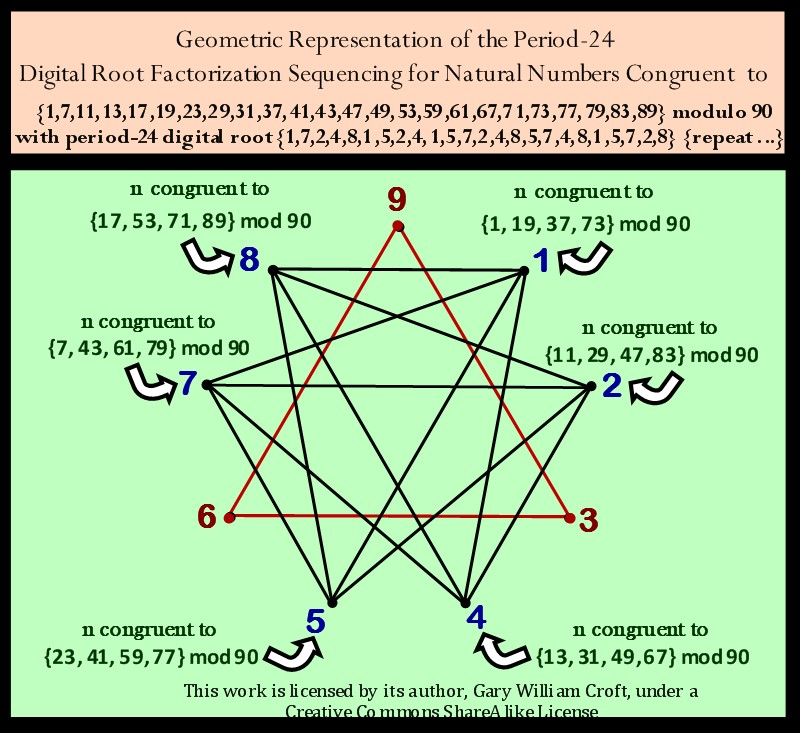Factorization with the Prime Spiral Sieve

Prime Number Sieving Algorithms | Digital Root Cycling | Modulo 90 Magic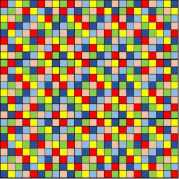This work is licensed by its author, Gary William Croft, under a Creative Commons Attribution-ShareAlike 3.0 Unported License

Factorization Dynamics

"Any time you hear about a striking result in number theory, there's a high chance that it's really a statement about modular forms." – Shamit Kachru, Ph.D., Stanford University | Institute for Theoretical Physics.

In this section we will dive deeply into the stunningly beautiful reflectional, rotational and palindromic mirror symmetries that are fundamental to the Prime Spiral Sieve's factorization algorithms when grouped modulo 90. Integral to these symmetries are 24 digital root dyad initiated period-24 cycles. These work combinatorially with mod 90 congruence relations to palindromically rotate the vertices of 242 = 576 {9/3} star polygons. The rotational sequences are encoded in 3x3 matrices. Each {9/3} star polygon generated, of course, possesses 3 equilateral triangles and 9 vertices. One complete factorization cycle, therefore, equates to 9 x 576 = 5184 vertice rotations. (The numbers 24, 576 and 5184 will figure large as we progress through this section.)

[Note: Here's a link to a paper describing these geometries published in the September, 2018 edition of the online Journal of Vedic Mathematics: "From Vedic Square to the Digital Root Clockworks of Modulo 90 Factorization".]

Regardless, what's important to keep in mind is that this elegant, efficient, deterministic methodology, rooted in perfect symmetry, leaves in its wake all prime numbers >5.

Prime factorization denotes the decomposition of a composite number into its non-trivial divisors, the product of which equals the original integer. In our case we're not referring to the 'top-down' prime factorization tree or trial division method you likely learned in high school. Rather, we're presenting a 'bottom-up' aproach using algorithms that deterministically project the locations within a spiral or on a matrix of all composite numbers and their not-necessarily-prime factors (meaning, every number populating the domain, with the exception of the number "1", is a 'factor' within the domain, regardless of primality). Ipso facto, Every composite number within the factorization domain is reducible to one or more 'factor dyads' found in the domain.

Chordal Progressions

Examining the Prime Spiral Sieve factorization sequences that index all composite numbers not divisible by 2, 3, or 5, we find multiplicative and additive-sum step algorithms that function in series-parallel. There is zero computational waste, i.e., no trial-and-error used whatsoever. Every composite number in the domain is located with absolute precision–and with relatively high number-crunching avoidance, aka efficiency. The algorithms in question generate period-8 'multiplicative group' structures (much like guitar chords on an 8-string guitar) that replicate clone-like along an 8 x n matrix (our virtual guitar's infinitely long fretboard).As an example, here's a graphic showing the first two 8-note chords generated by 7 (the first sequence in a progression generated by numbers congruent to {7} modulo 30):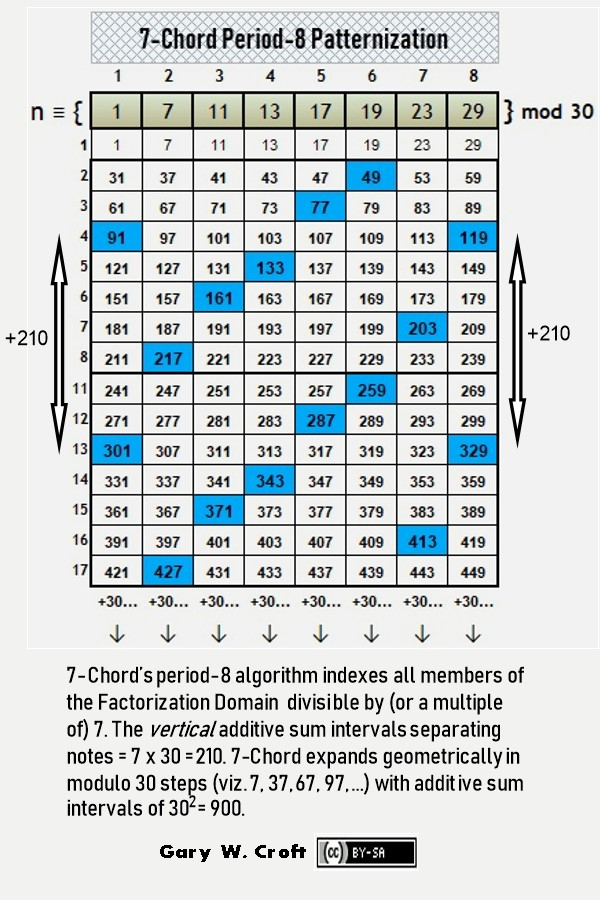Staying with our example, here the chord for n ≌ {7} mod 30 expands to n ≌ {37} mod 30, illustrating how the chords stretch with each modulo 30-interval step, while maintaining their relative positions: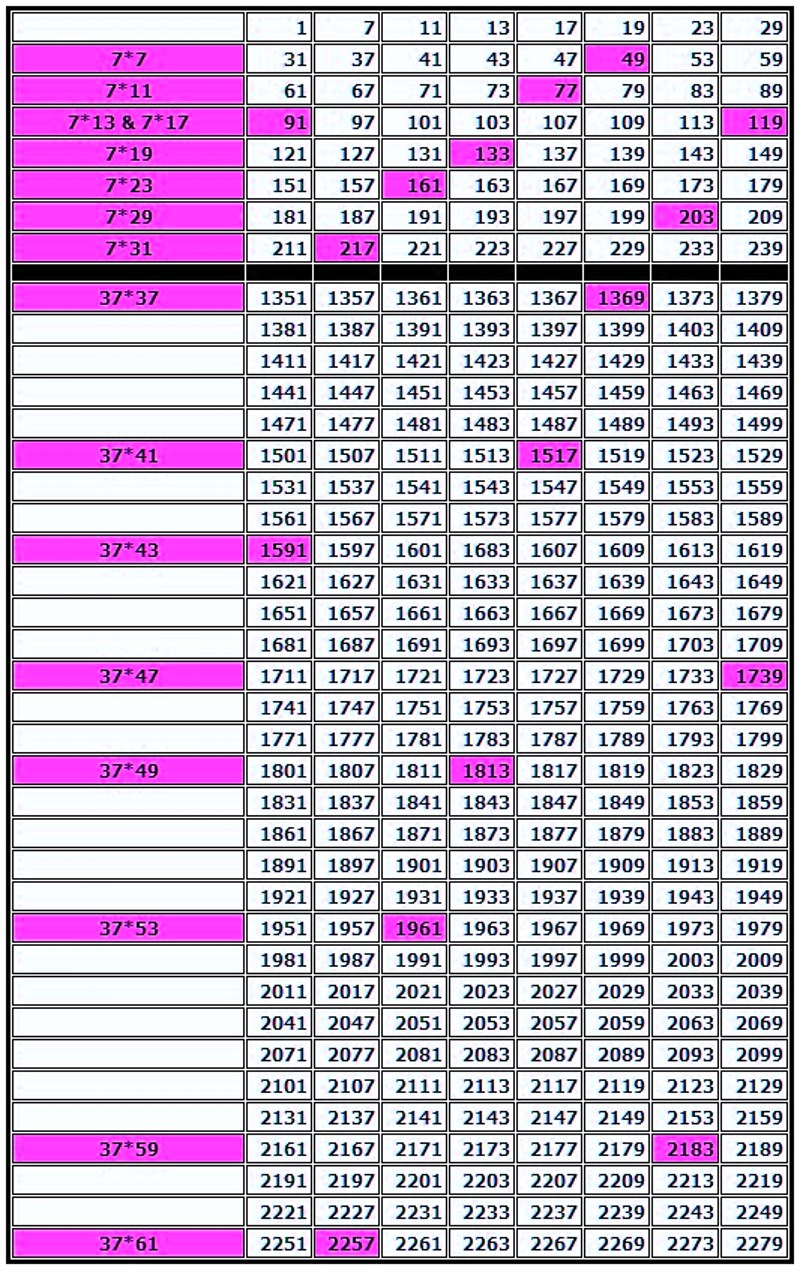And here we detail the calculations that generate the first four chords for numbers 7, 11, 13, 17, 19, 23, 29, 31, and 37 (the first number in our domain (1) being 'silent'):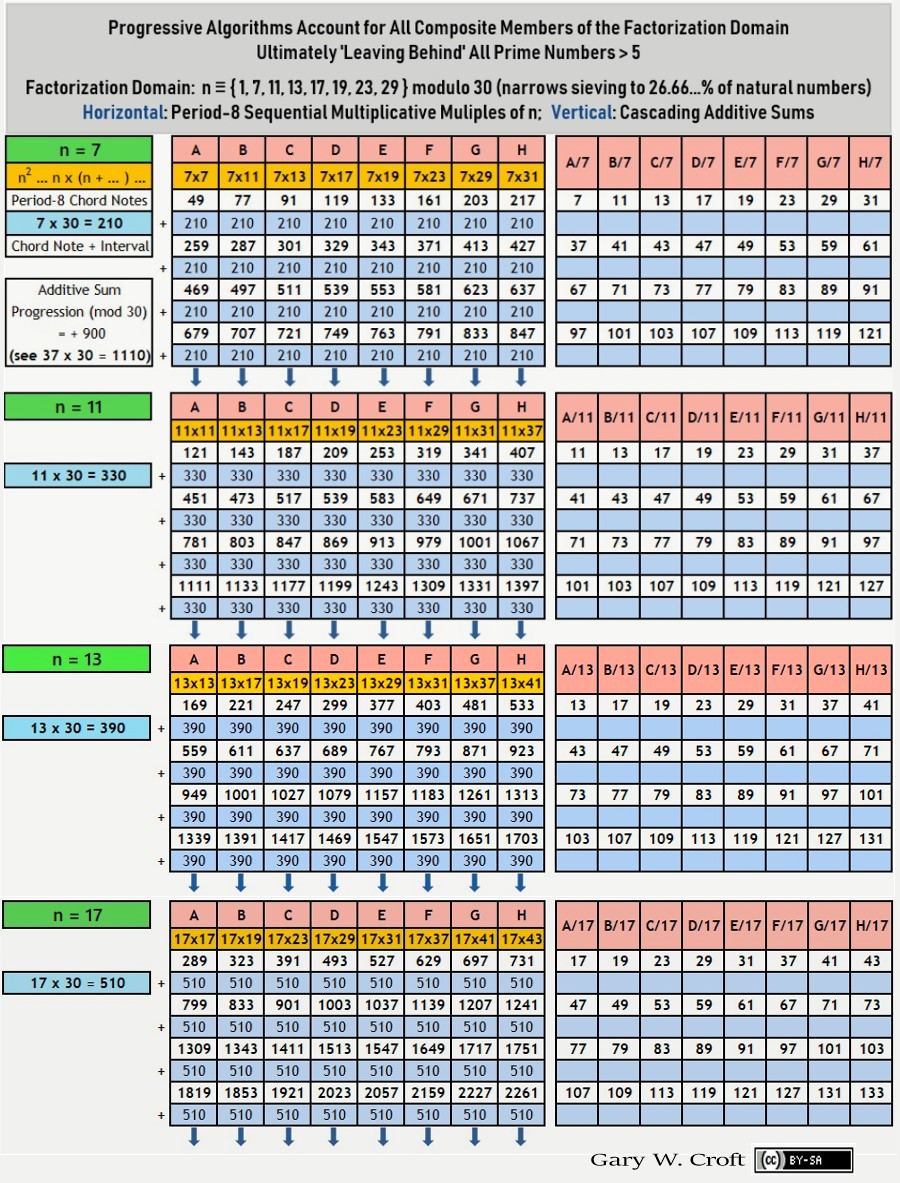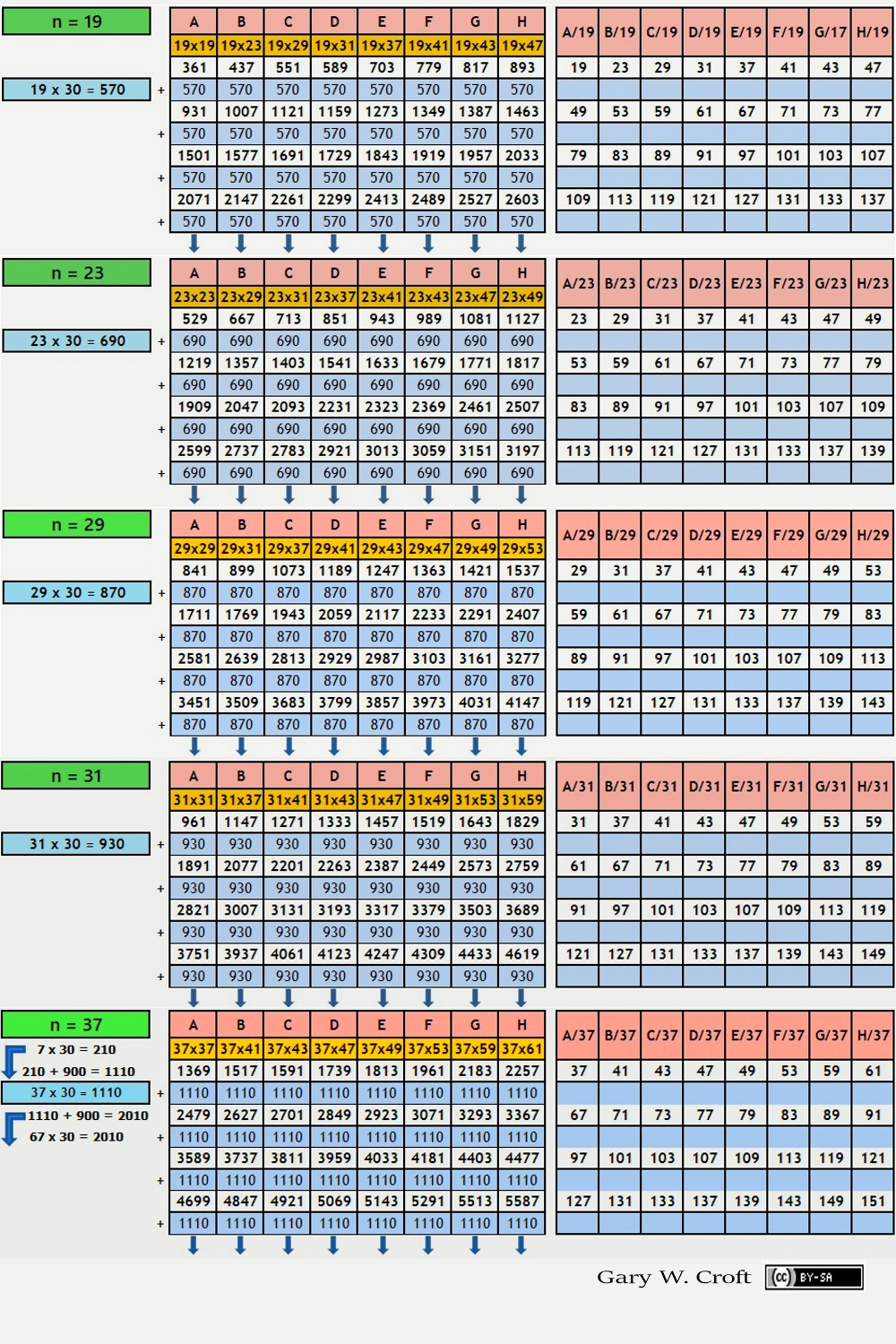To view a color-coded matrix demonstrating the results of factoring one complete chord progression, click here. Because the 8-chord/64-note sequences overlap, with the products from the next sequence's factorizations beginning before the prior sequence ends, an illusion is created of chaos and randomness. The reality, however, is that the eight chord sequences factor and progress geometrically with deterministic precision and unvarying symmetry when understood as independent albeit superimposed mathematical objects.

We must note here–and this will become amplified, below–that the power of the above described period-8, modulo 30 algorithms is leveraged significantly when their frame is tripled to create period-24 chords, modulo 90 (which expands the formula for intervals from n x 30 to n x 90). Given that our domain has a period-24 digital root, we can then parse the results by digital root. To illustrate, the graphic below shows the first 11-Chord, framed period-24, modulo 90. It would be an easy matter to 'hide' columns and sieve by any combination one would choose. For example, knowing that all twin prime candidates greater than (5,7) must have digital root sequencing of either {2,4}, {5,7}, or {8,1}, we can easily determine the impact of 11-Chord sequencing on any combination of twin prime digital root variations we choose.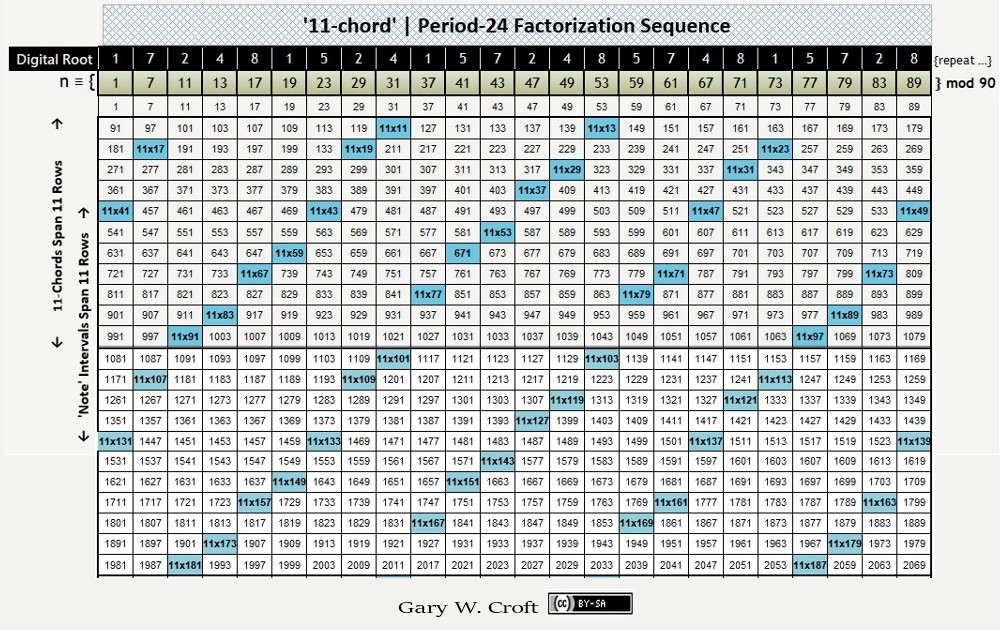Factorization Algorithms

The 'chords' described above lay the foundation for three basic ways to algorithmically identify composite numbers in our factorization domain (natural numbers not divisible by 2, 3 or 5): the first employs multiplication, the second division, and the third and most complex uses all four of the fundamental operations of arithmetic: multiplication, division, addition, and subtraction:

1. All composite numbers in the array are the products of all preceding numbers in the sequence times themselves and all subsequent numbers. The one exception is 1, which effectively self-eliminates (1x1 = 1 tags itself as a non-prime). Thus (using '7-chord' as an example): 72 tags 49 as a composite; 7x11 tags 77 as composite ... an infinite string.

Below is an interesting graphical array of these products (composite numbers):2. The array can be efficiently factorized using modular arithmetic by simply dividing the set unto itself sequentially: All modulo n ≌ 0 results generated (except when a number is divided by itself) indicate factors (arrayed horizontally, hi-lited in blue) of n (arrayed vertically, hi-lited in yellow), as illustrated below.

[Note: Where there's only one zero, n is a square. If your intent is to simply determine the primality of a specific number (for example number 209 in the table below), given that 209 can't have a prime factor greater than its square, and that the square root of 209 is 14.45..., one need only test this series to mod 13 since encountering one zero identifies a number as composite. And, speaking generally, to make the number-crunching even more efficient the sieving program can be coded to bypass retesting numbers already proven to be composite.]: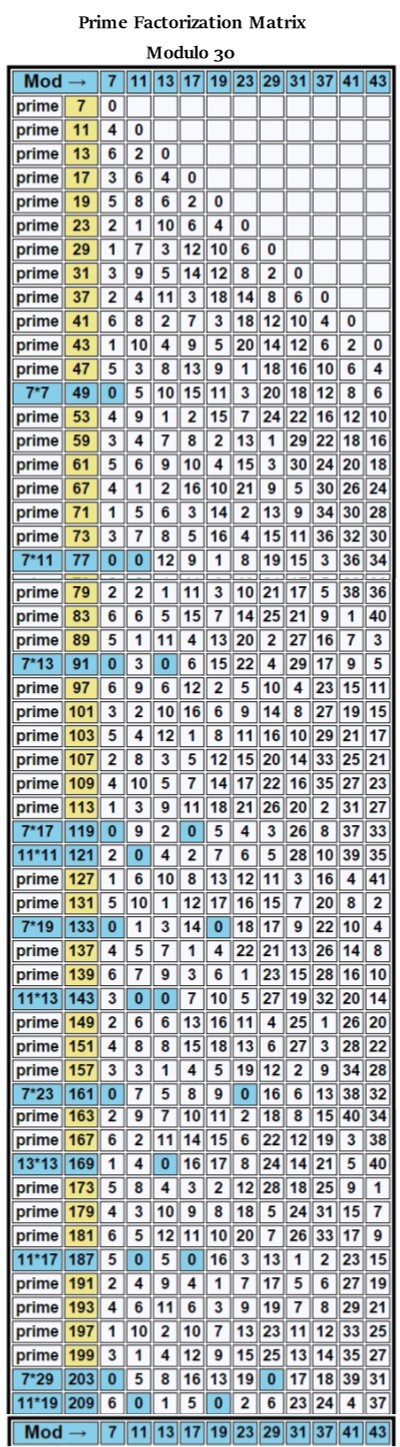1. The third factorization method is cumbersome and inefficient. However, because it employs the root prime angles, in a procedure involving multiplication, alternating addition or subtraction, as well as division to derive factors, it is nonetheless interesting for its own sake, and shows promise of opening new lines of inquiry. If this piques your curiosity, click here.

[Note: If you use Python (the computer programming language designed for the development of scientific, engineering and mathematics applications), an MIT licensed Python module dubbed "pyprimes" designed to run and compare prime number sieving algorithms, including the Prime Spiral Sieve (using its former name, "Croft Spiral"), can be found here or here at code.google.com The programmer, by-the-way, rates the Prime Spiral Sieve "fastest/best" and recommends it as "the preferred way of generating prime numbers" compared to several sieves tested.]

Digital Root Multiplication Dyads: The 36 Fundamental Operations of Modulo 30 Factorization

"Vedic Mathematics is ... a sophisticated pedagogic and research tool ... ." – Dr. L.M. Singhvi, former UK High Commisioner for India, from his forward to Cosmic Calculator, by Williams & Gaskell

To gain a deeper understanding of the algorithms driving the factorization and prime number sieving methods described above, we will venture into the realm of digit sum arithmetic. By analyzing the sequencing of digital root elements belonging to this set, we will discover the fundamental patterns underlying all factorizations generated by this sieve.

First, let's define our terminology, which–please note–may vary slightly from how it's used elsewhere: digit sum is simply the sum of a number's unit values, e.g., 49 = 4+9 = 13 = digit sum. digital root (aka "repeated digit sum," "iterated sum-of-digits," or "modulus 9 function") refers to the single digit (1-9) resulting when all digit sums are summed in turn, e.g., 49 = 4+9 = 13 = 1+3 = 4 = digital root. Digital roots will be expressed in this form: dr(n). When digital roots are multiplied, the product is expressed in its digital root form, thus dr(2) x dr(5) = dr(1), given that 2x5=10 and the digital root of 10 is 1, or dr(1).

Analyzing the factorization algorithms discussed thus far from a digital root perspective leads us to the 6 x 6 = 36 fundamental operations of modulo 30 factorization. Below is a Vedic Square, an ancient digital root multiplication matrix attributed to Indian mathematicians. We've 'lightened' it by removing its 3's, 6's and 9's. This was done to reflect the fact that our modulo 30 factorization domain is restricted to digital roots 1, 2, 4, 5, 7, and 8 (and we note that technically the subset {1, 2, 4, 5, 7, 8} forms a cyclic group of multiplicative units in the ring Z/9Z, forming the 6 x 6 = 36-cell Latin Square below we've dubbed 'Vedic Square Light'). Given all of the diagonals are palindromic, the matrix has reflectional symmetry. Another dimension of symmetry: every row and column consists of 3 bilateral-9 sums: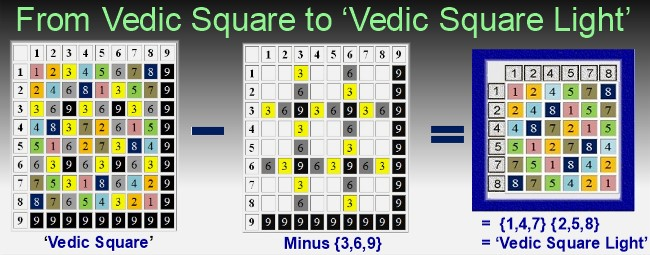Reconfiguring our 'Vedic Square Light' to parse the factor dyad products by digital root gives us the 36 fundamental digital root factorization dyads for all composite numbers in our modulo 30 defined domain: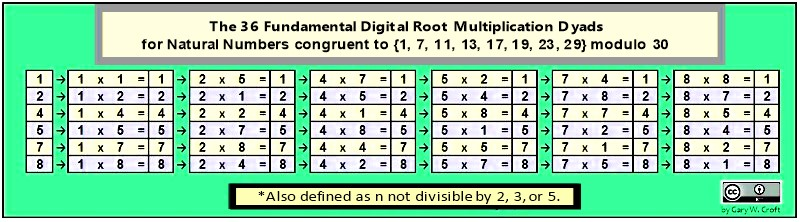Can you find this 36-digit palindrome weaving through the matrix above like two opposing square waves?:
1, 2, 4, 5, 7, 8, 4, 8, 7, 2, 1, 5, 7, 5, 1, 8, 4, 2, 2, 4, 8, 1, 5, 7, 5, 1, 2, 7, 8, 4, 8, 7, 5, 4, 2, 1

The set of natural numbers not divisible by 2, 3 and 5 when arrayed in 8 columns has an infinitely repeating digital root sequence expressed in one of two sets: {1,4,7} or {2,5,8}, albeit the starting digit varies. The illustration directly below shows the repeating digital root sequences that radiate along the 8 spiral sieve diagonals; also shown are the digital root sums of adjacent diagonals (in yellow).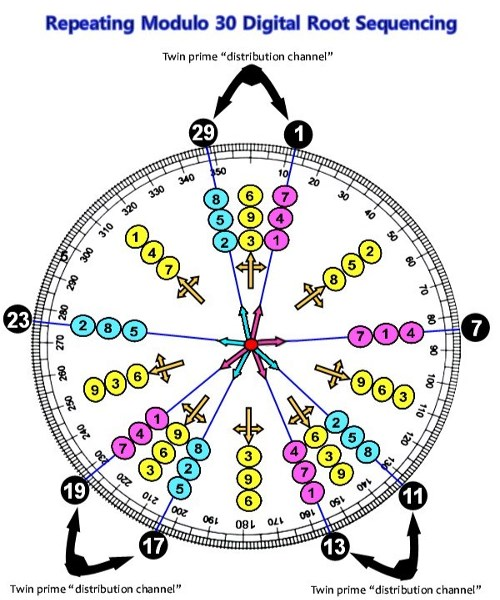The {1,4,7},{2,5,8} and {3,6,9} repeating triplets are known as the "Trinity of Triangles" that originate when constructing a 9-point star in the form illustrated below (also pictured, at the center of the star, is perhaps the best known magic square of all, attributed to Lo Shu, incorporating the numbers 1 thru 9 where all rows, columns and principal diagonals sum to 15). Also pictured are the corresponding "Trinity of Magic Squares" (technically, Latin Squares) which in combination reveal their own {3,6,9} triangular digital root sequencing.

[Note: To see how the Lo Shu Magic Square and Trinity of Magic Squares can be combined in a "4-Fold Way" to create their own magic square when configured in a 4 x 4 matrix of magic squares consisting of 144 (122) elements where all rows, columns and principal diagonals sum to 60 and the sum total of the matrix's elements = 720 = 360 x 2, click here]: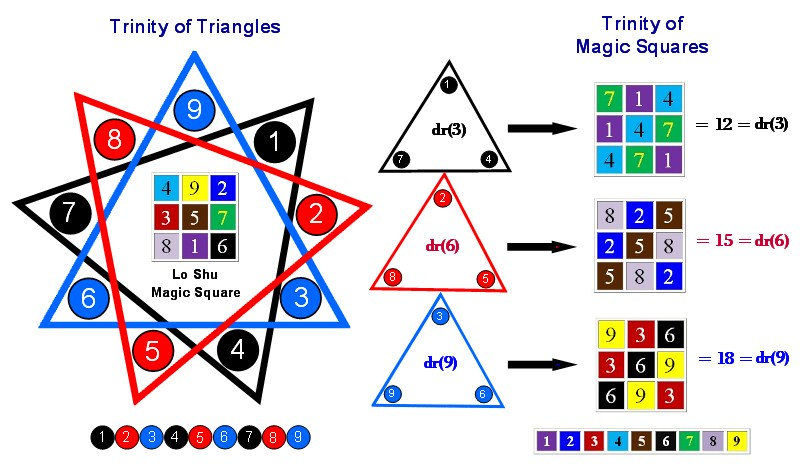Like a coin sorter, below we parse the first 41 elements of our domain as they naturally sequence under digital root dyads {2,4} ... {8,1} ... {5,7} (albeit with algorithmically determinable gaps). This range starts with the number 1, and includes 33 prime numbers (10 pairs of which form twin primes) and 7 composite numbers (for which we display both natural and digital root factors):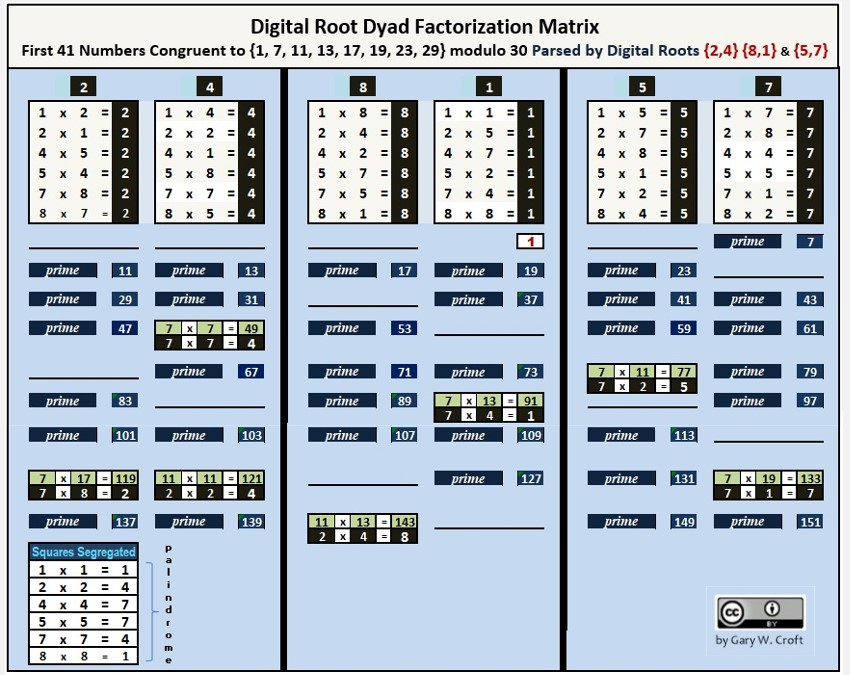Modulo 90 Congruence Matrix

The matrices below reveal that the factorization algorithms generated within our domain possess perfect symmetry when the products are converted to their modulo 90 congruence and framed by their period-24 horizon. For example, 7 x 17 = 119 which is congruent to 29 (modulo 90). On the matrix below you will find that n ≌ 7 mod 90 x n ≌ 17 mod 90 = n ≌ 29 mod 90. And note that the additive sum of all columns and rows = 1080 = 360 x 3, while every row and column consists of 12 bilateral-90 sums and 12 bilateral-10 terminating digit sums, and all diagonals are palindromic.

Below is the matrix, a beautiful Latin Square. We conjecture that it represents an optimal factorization 'packing' algorithm akin to "Optimal Sphere Packing in Dimension 24." [Note: Down this page, we completely "unpack" this algorithm that efficiently compacts 242 = 576 factorization dyads.]

Aside from its perfect palindromic mirror symmetry and algorithmic beauty, this matrix is the source of four proofs (and we can surmise others): 1) The squares of all prime numbers greater than 5 must be congruent to {1, 19, 31, 49, 61, or 79} modulo 90, and therefore; 2) the squares must have a digital root of 1, 4, or 7, and conversely; 3) the squares (including the squares of all primes >5) can't possibly be congruent to {7, 11, 13, 17, 23, 29, 37, 41, 43, 47, 53, 59, 67, 71, 73, 77, 83 or 89} modulo 90; 4) nor can they have a digital root of 2, 5, or 8 (joining 3, 6, and 9):Two of the palindromic diagonals in the above matrix demand special attention: For one, the period-24 principal diagonal (upper-left to lower right hand corners, color-coded blue) captures the modulo 90 congruence of all the perfect squares in our factorization domain. In modulo 90 terms they are:

n ≌ {1, 49, 31, 79, 19, 1, 79, 31, 61, 19, 61, 49, 49, 61, 19, 61, 31, 79, 1, 19, 79, 31, 49, 1} mod 90

... which reduces to:

n ≌ {1, 19, 31, 49, 61, 79} modulo 90

... which in turn is a subset of the twin prime candidate distribution sequence for all twin primes > (5,7):

n ≌ {1, 13, 17, 19, 29, 31, 41, 43, 47, 49, 59, 61, 71, 73, 77, 79, 89} modulo 90.

This construction proves that the squares of all natural numbers not divisible by 2, 3 or 5 (which includes all prime numbers >5) must be congruent to either 1, 19, 31, 49, 61, or 79 modulo 90 and thus must have a digital root of either 1, 4, or 7, and a terminating digit of 1 or 9. Conversely, members of this domain with digital root of either 2, 5, or 8 cannot be perfect squares. This matrix also proves that every 'beat' of the sequence of squares negates a twin prime candidacy, the first 'casualty' being (47 [prime], 49 = 72).

The other palindromic sequence of special interest is the secondary diagonal (lower-left to upper-right hand corner; color-coded red). It consists exclusively of prime numbers and is point-counterpoint to the sequence of modulo 90 squares, detailed above:

n ≌ {89, 41, 59, 11, 71, 89, 11, 59, 29, 71, 29, 41, 41, 29, 71, 29, 59, 11, 89, 71, 11, 59, 41, 89} mod 90 {repeat …}.

It reduces to prime numbers {11, 29, 41, 59, 71, 89} x 4 = 1200 with digital roots restricted to 2, 5, and 8 (and note 11+89=100; 29+71=100; 41+59=100).

The sequence can also be derived formulaically from the squares of n ≡ {1, 7, 11, 13, 17, 19, 23, 29} mod 30, noted above (framed mod 90; period-24). Here’s the formula:

a(n) = 90 − ( n ≌ {1, 7, 11, 13, 17, 19, 23, 29}(n)2 mod 90).

The first 9 terms in this sequence compute to:

For n = 1: k = 90 − mod 90 of ^2 gives k = 89;
For n = 7: k = 90 − mod 90 of 2 gives k = 41;
For n = 11: k = 90 − mod 90 of 2 gives k = 59;
For n = 13: k = 90 − mod 90 of 2 gives k = 11;
For n = 17: k = 90 − mod 90 of 2 gives k = 71;
For n = 19: k = 90 − mod 90 of 2 gives k = 89;
For n = 23: k = 90 − mod 90 of 2 gives k = 11;
For n = 29: k = 90 − mod 90 of 2 gives k = 59;
For n = 31: k = 90 − mod 90 of 2 gives k = 29...

Summing the principal and secondary diagonals gives us 1200 + 960 = 2160 = 360 * 6 = 432 * 5. And aligning the principal and secondary diagonals forms this string of 24 dyads summing to 90 each, again for a total of 2160 (and note that only terminating digits 1 and 9 are present and that there are also 24 diagonal dyads summing to 90 each, as somewhat crudely illustrated):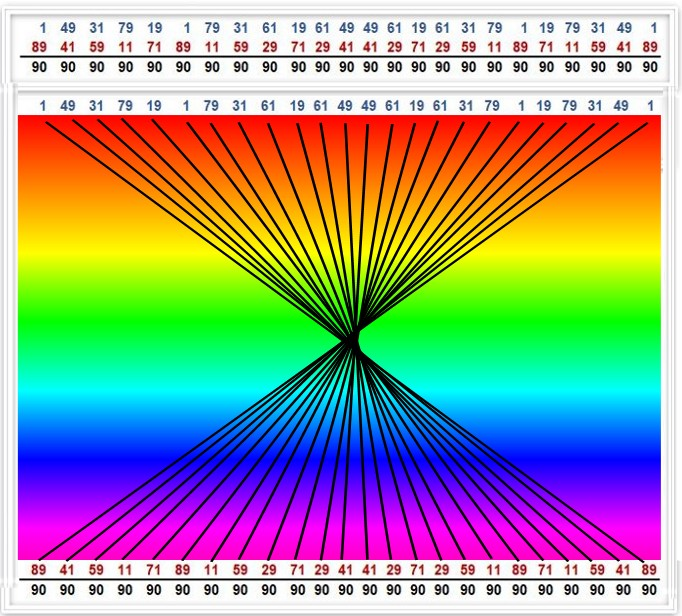Next, we provide two examples of the 24 sets of 24 multiplicative dyads, highlighting the products of the 24 dyads that produce results congruent to {1 and 71} modulo 90, followed by lists of the dyads themselves. And we note that the sets of 24 each n congruent to {1 and 71} modulo 90 dyads display perfect end-to-end bilateral 90-sum symmetry which translates to perfect 9-sum symmetry at the digital root level (and this holds true for all 24 sets). [Here's a link to an Excel spreadsheet that documents all 24 sets of 24 factorization dyads. And they are presented in tables further down this page, as well.]

Study these, and you'll come to the profound realization that the 24 sets can be visualized end-to-end as a spiral with 288 counter-balancing 90-sums on the multiplier side and rotational mirroring on the multiplicand side for another 288 = 122 x 2 bilateral 90 sums. This 24-fold concatenation of symmetries at the heart of nature is truly awe inspiring, especially when you consider that they ultimately determine the distribution of all prime numbers > 5 (while the first three primes {2, 3, 5} and their primorial 30 construct the scaffolding within which the algorithmically generated factorization dyads operate):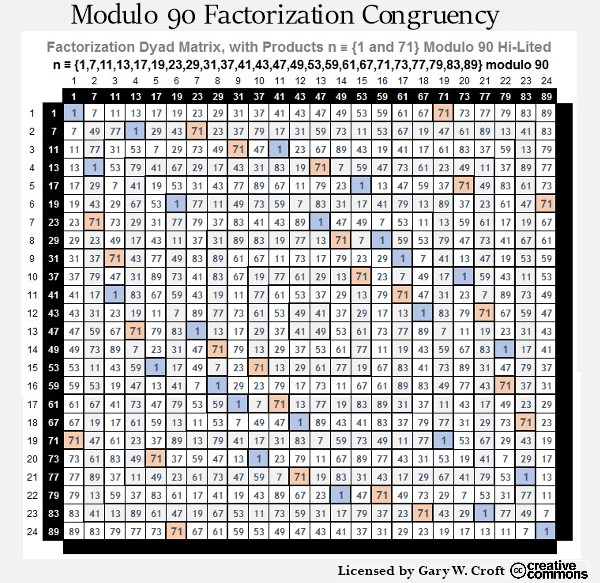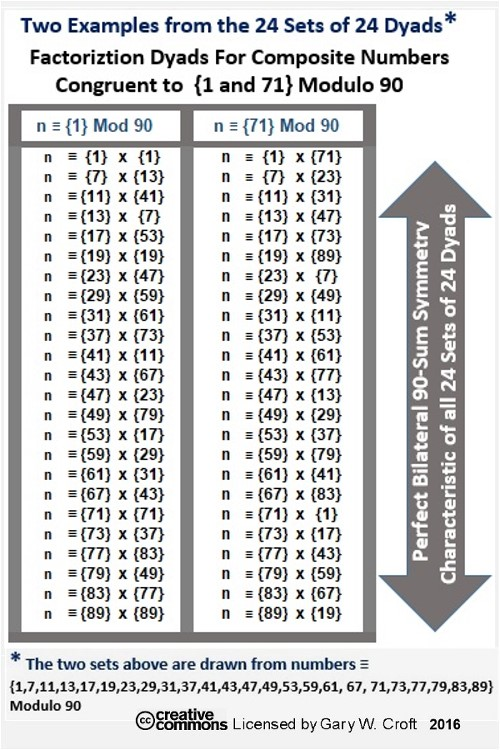The 24 factorization dyads listed above that account for all composite numbers congruent to {1} modulo 90 give one pause when you consider that there are exactly 1000 prime numbers between 1x1 and 89x89. And we remind you that 89 is the 24th prime number and the 24th natural number not divisible by 2, 3, and 5, and that 242 = 576, the total number of factorization dyads in our modulo 90 defined domain (arrayed in the tables that follow). And we find it astonishing that 82 x 9 = 576 and 82 x 92 = 5184 = 12 x 432 = 9 x 576 (the number of vertice rotations in one complete 242 = 576 factorization cycle).

And note that 5 * 7 * 6 = 210 = the first 4 primorial primes: 2 * 3 * 5 * 7 and that 12 * 22 * 32 * 42 = 576. In addition, when we take the digits of the 1000th prime number, 7919, and multiply them together we get 7 * 9 * 1 * 9 = 567 and 5 * 6 * 7 = 210 = 2 * 3 * 5 * 7. Then note that 7919 is the 2112th natural number not divisible by 2, 3, or 5 and that 11 * 576/3 = 2112. Another interesting feature of 576 is that the cumulative digital root for natural numbers 1, 2, 3 ..., i.e., {1 + 2 + 3 + 4 + 5 + 6 + 7 + 8 + 9} {repeat ...}, from 1 to 576 = 2880 = 8 * 360 = 5 * 576; and 576 + its cumulative digital root 2880 = 3456 and 3 * 4 * 5 * 6 = 360.

Also, the cumulative digital root of 24 = 111 (the 3rd repunit, R3); and 111 = (1 * 102) + ( 1 * 10) + (1 * 1). And we note that 24 is the 3rd 'Superprimorial,' viz. 1! x 2! x 3! = 24. And then there's this: F24 = 46368 and 4 x 6 x 3 x 6 x 8 = 3456; and, (3+5) x (4+5) x (5+5) x (6+5) = 7920 = 22 x 360; and, (3+5) x (4+5) x (5+6) x (6+6) = 9504 = the cumulative digital root sum of n not divisible by 2, 3, or 5 for the range (1-7919). Speaking of 3456, later, we'll be discussing the role of the 5th Superprimorial, 34560, and it's relationship to 7919. And, we'll take a deep dive into 'a number of numbers' such as these that behave like mathematical objects in-and-of themselves, as they form beautiful structures that determine the distribution of prime numbers.

The tables below account for all 24 sets of 24 dyads: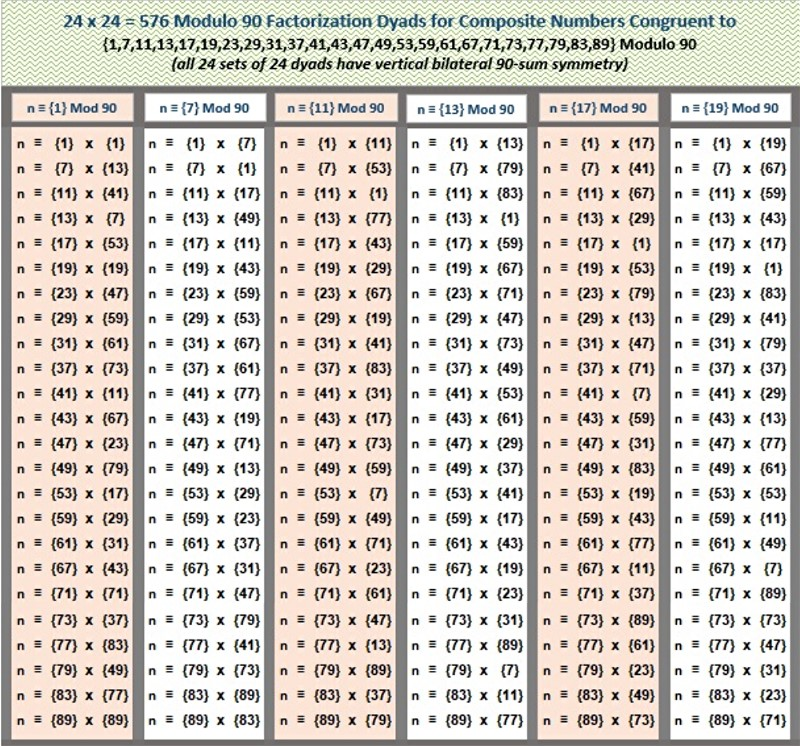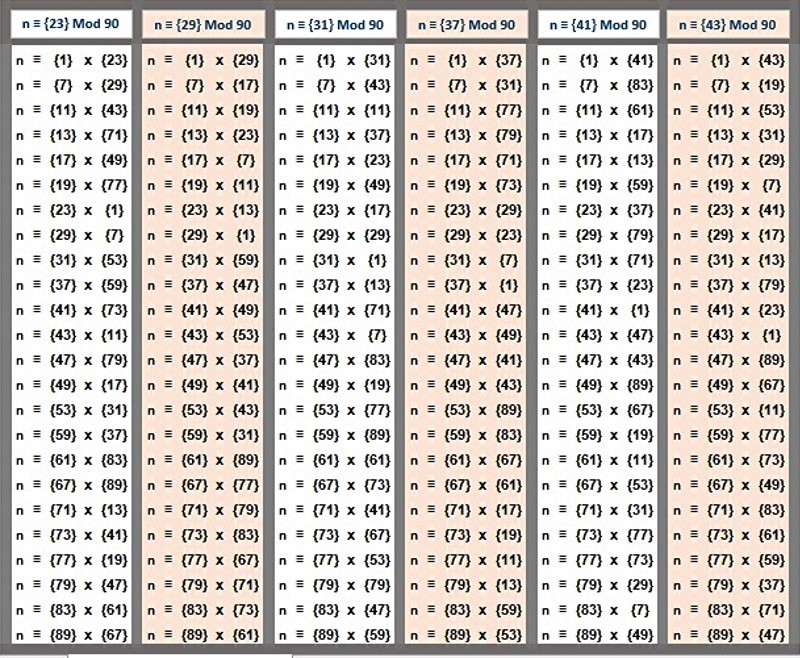Reflecting back on the 6 x 6 = 36 fundamental digital root operations of modulo 30 factorization, below we've organized the 576 modulo 90 factorization dyads into 36 sets of 16 each, parsed by digital root dyads. The result–a factorization clockworks–is profoundly beautiful, especially given these dyads combinatorialy generate period-24 cycles that codify the construction of 576 {9/3} star polygons (discussed and illustrated in detail down this page under the heading, "Period-24 in All Directions.").

We conjecture that these constructions are isomorphic, if not manifold, to what is known as Equilateral Triangle Lattice Universal Optimality (for which there is considerable interest in finding a proof). The 24-dimension algorithmically constructed symmetry groups described here involving the rotating vertices of equilateral triangles within {9/3} star polygons provide both the 24- and 9-dimensional geometries needed for a proof. This is related in turn to Maryna Viazovska's R24 optimal sphere packing theorem, referenced below (with a link to her paper) in the section headed "Seeing Stars: Rotational Symmetry Groups, Modulo 90."Modulo 90 Digital Root Multiplication/Factorization Matrix

Below is a modulo 90 multiplication matrix with the products converted to their digital roots that forms a diagonally palindromic Latin Square. It can be used in conjunction with the factorization matrices pictured above. The principal diagonal has been colored green to differentiate the squares.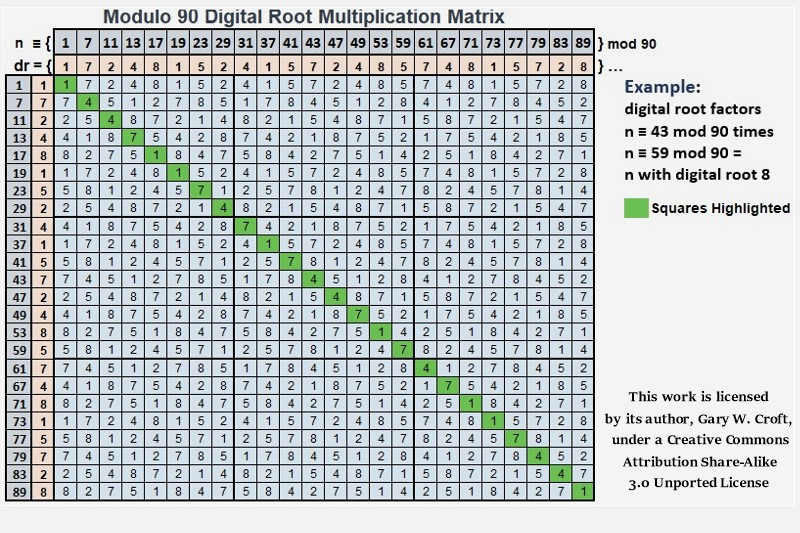Studying the digital root of the squares highlighted in the matrix above we discover a period-24 palindrome,
{1, 4, 4, 7, 1, 1, 7, 4, 7, 1, 7, 4, 4, 7, 1, 7, 4, 7, 1, 1, 7, 4, 4, 1} {repeat ...},
and find the basis for a proof that the squares of all n ≌ {1, 7, 11, 13, 17, 19, 23, 29, 31, 37, 41, 43, 47, 49, 53, 59, 61, 67, 71, 73, 77, 79, 83, 89} modulo 90 (and therefore by definition all prime numbers > 5) distribute to digital roots 1, 4, or 7, categorized in three sets as follows:

n ≌ {1, 17, 19, 37, 53, 71, 73, 89}2 mod 90 = digital root 1
n ≌ {7, 11, 29, 43, 47, 61, 79, 83}2 mod 90 = digital root 4
n ≌ {13, 23, 31, 41, 49, 59, 67, 77}2 mod 90 = digital root 7

And note that all three sets have four bilateral 90-sums = 360. We've documented this sequence on the On-Line Encyclopedia of Integer Sequences; Ref: OEIS Sequence Number A240924.

Subsorting (sieving) the squares of twin prime candidates gives us:

n ≌ {1, 17, 19, 71, 73, 89}2 mod 90 = digital root 1
n ≌ {11, 29, 43, 47, 61, 79}2 mod 90 = digital root 4
n ≌ {13, 31, 41, 49, 59, 77}2 mod 90 = digital root 7

Each of the three sets in this sub-division has three bilateral 90-sums = 270.

And here we've color-coded the matrix to highlight digital root distribution symmetries: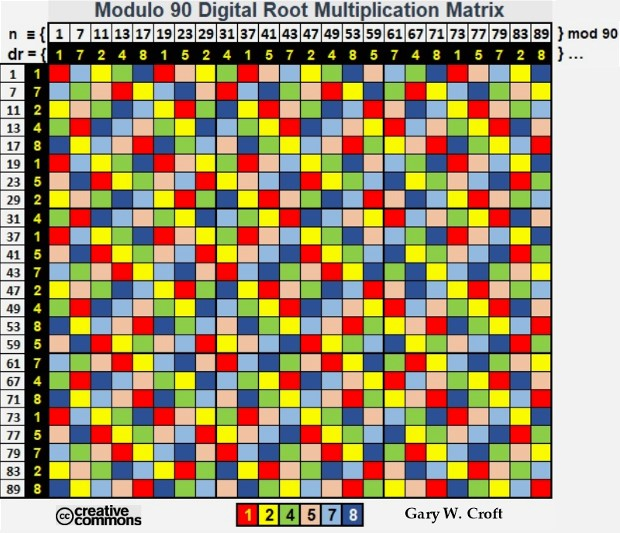The terminating digits of the factorization dyads we've been enumurating also have organizing principles that generate symmetry, as illustrated below (note the 16-digit palindrome):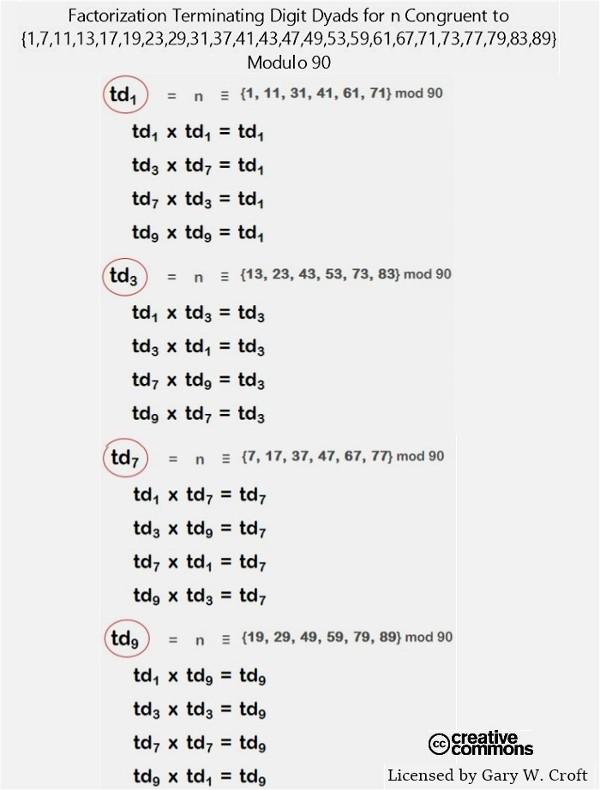These symmetries can be traced to the period-24 terminating digit repetition cycle of our domain, each repetition possessing 12 bilateral-10 sums:

{1, 7, 1, 3, 7, 9, 3, 9, 1, 7, 1, 3, 7, 9, 3, 9, 1, 7, 1, 3, 7, 9, 3, 9} { repeat ... }

The simplest way to expose terminating digit symmetries is to matrix multiply them, as pictured below: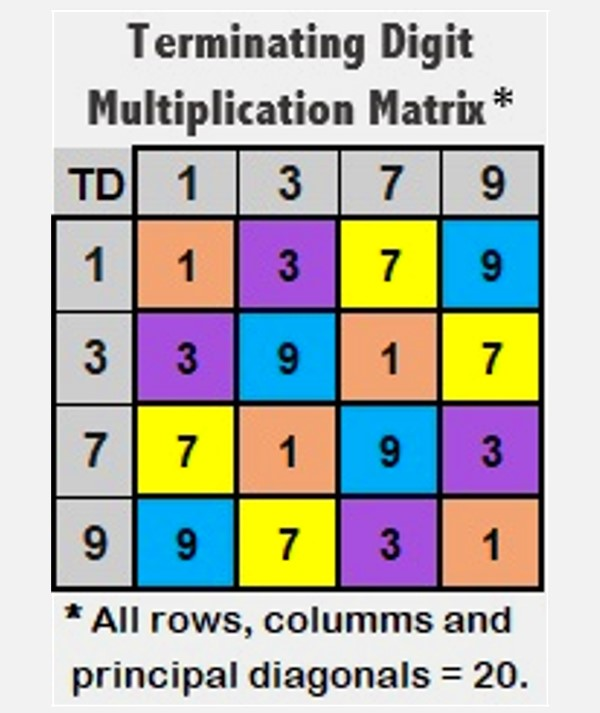Modulo 90 Factorization: Congruence Dyad Matrix

A fascinating dimension of numbers ≌ {1, 7, 11, 13, 17, 19, 23, 29, 31, 37, 41, 43, 47, 49, 53, 59, 61, 67, 71, 73, 77, 79, 83, 89} modulo 90 is that modulo 90 congruence can be determined without division for any member of this sequence by matrixing a number's digital root with its terminating digit (see illustration, below). For example, take the number 389: Its digital root = 2 and its final digit = 9. Referencing the matrix below, we see that 389 is congruent to 29 mod 90. [And once again we discover an object with 90-sum symmetry.]All Factor combinations for composite numbers within our modulo 90 defined domain can be rooted to one or more 'modular congruence dyads' drawn from the 24 candidates shown in the table above.

It can be easily proven that six of the digital root / terminating digit dyads in the matrix above classifying members of our domain cannot possibly be twin prime candidates for pairs > (5,7), given their closest proximity to other primes is ±4:

dr1...td7 (n ≌ {37} mod 90)
dr2...td3 (n ≌ {83} mod 90)
dr4...td7 (n ≌ {67} mod 90)
dr5...td3 (n ≌ {23} mod 90)
dr7...td7 (n ≌ {7} mod 90)
dr8...td3 (n ≌ {53} mod 90)

[Note: To quickly determine a number's modulo 90 congruence, reduce all digits preceding the number's terminating digit to their digital root. For example, the 1st three digits of 3797 reduce to digital root 1 leaving 17, and thus 2497 ≌ {17} mod 90. Remember that 9's are 'cast out' when calculating digital root.]

The Prime Spiral Sieve as Star Birthing Chamber

Rotating Equilateral Triangles, Triangulation Mapping Matrices, Complex Polygons and the Digital Root of Modulo 90 Factorization

"The search for symmetry, and the emotional pleasure we derive when we find it, must help us make sense of the world around us ... Symmetry is also economy. Symmetry is simplicity. Symmetry is elegance." From The Accidental Universe, by theoretical physicist Alan Lightman.

Seeing Stars: Rotational Symmetry Groups, Modulo 90

When we stretch our modulo 30 ↔ 8-dimension horizon times 3, and look at the above factorization sequences from a modulo 90 ↔ 24-dimension digital root perspective, we make a profound discovery (illustrated in the graphics that follow): Straight out of group theory comes a triad of rotating equilateral triangles, ({1,4,7}, {2,5,8}, {3,6,9}), algorithmically permutated to produce 242 = 576 {9/3} star polygons per one complete cycle, which equates to 242 x 3 = 1728 rotating equilateral triangles possessing a total of 9 x 576 = 5184 vertices (And we think it worth repeating that there's an amazing connection between 89 and 5184 in that there are exactly 1000 prime numbers between 1 and 892 and 82 * 92 = 5184, not to mention 82 * 9 = 576!).

Each star polygon's sequence of rotations is digitally rooted in a 3x3 matrix that communicates rotational instructions to the star's vertices. These digital root sequences and their associated geometries are infinitely repeating (modulo 90), and the symmetries they create are reflectional, rotational and translational.

It's clear that the factorization algorithms for numbers not divisible by 2, 3, and 5 are complex polygon generators. Put more poetically perhaps, the Prime Spiral Sieve, when telescoped x 3 to modulo 90, is a star birthing chamber. These symmetry groups are digitally rooted in numbers ≌ to {1, 7, 11, 13, 17, 19, 23, 29, 31, 37, 41, 43, 47, 49, 53, 59, 61, 67, 71, 73, 77, 79, 83, 89} modulo 90, and form the basis of the 24 x 24 "Magic Mirror Matrix" (see also section below titled "Cirque de Primes Juxtaposed with the Magic Mirror Matrix").

Mathematicians often compare prime numbers to 'atoms' and composite numbers to 'molecules.' One could argue that it's consistent with this analogy to call digital root equilateral triangles {1,4,7} {2,5,8} the 'quark triads' of the number universe. In both cases we have a fundamental dyad of interacting triads. With regard to our modulo 90 triangulations, {1,4,7} and {2,5,8}, each has 3! = 6 possible rotational symmetry operations referenceable to the values of vertices 1 thru 9 of a {9/3} star polygon; thus we get: {1,4,7}, {1,7,4}, {4,1,7}, {7,1,4}, {4,7,1}, {7,4,1} vs. {2,5,8}, {2,8,5}, {5,2,8}, {8,2,5}, {5,8,2}, {8,5,2} (which you'll note we've ordered palindromically). These triangles interact combinatorially via digital root dyad sums to create {3,6,9}'s six possible rotational permutations {3,6,9}, {3,9,6}, {6,3,9}, {9,3,6}, {6,9,3} and {9,6,3}, illustrated below: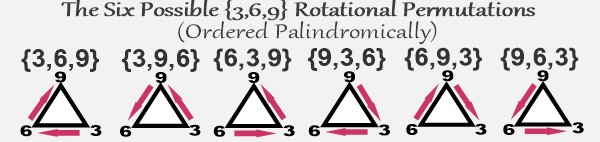As we've noted previously, the {3,6,9}'s triangular permutations provide fundamental structure to our domain in the form of repeating modulo 30, 60, 90 {3,6,9} cycles ... These progressions appear to be isomorphic to the geometric hierarchy of star polygons constructed from equilateral triangles such that (Schäfli notation in braces): {3/1}, or simply {3}, is to modulo 30 as {6/2} is to modulo 60 as {9/3} is to modulo 90; ... building to infinity. For example, along this sequence of star polygons you'll find {27/9} ... {360/120} ... {432/144} ... {576/192}, and {1080/360} ... ultimately converging to an annulus; Wikipedia definition: "a region bounded by two concentric circles."In much the same way that a Buddhist might say "It's the interaction between Yin and Yang that moves fate forward," we're arguing that it's the interaction between {1,4,7} and {2,5,8} that moves structure foward shaped by {3,6,9}'s six combinatorial symmetries. Writing about the importance of group theory developed by Évariste Galois, well known mathematician Marcus du Sautoy captures this idea in his book Symmetry: A Journey into the Patterns of Nature: "[Galois] discovered that it is the interactions between the symmetries in a group that encapsulate the essential qualities of the symmetry of an object."

With such symmetry interactions in mind, let's examine the fundamental operations at work involving the three equilateral triangles ({1,4,7} {2,5,8} {3,6,9}) that form {9/3} star polygons, the vertices of which are rotated by the modulo 90 factorization algorithms discussed in this section. The graphic below documents the set of 36 permutated Latin squares derived from the aforementioned vertices. The 36 sets of vertical and horizontal triads, along with primary and secondary diagonals, sum to 4,320 = 12 x 360. And note that 4,320 − (1092 − 892) = 360.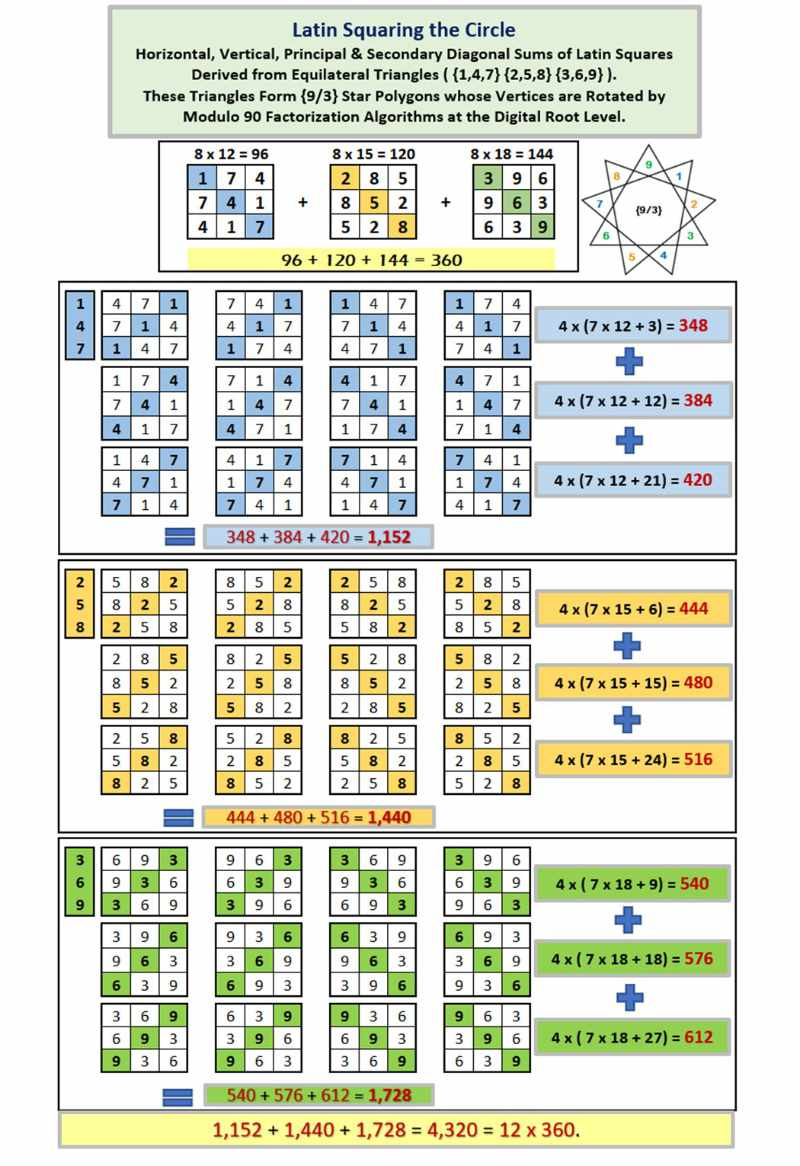[Note: Any argument for the significance of {3,6,9} must include genius inventor Nikola Tesla's famous quote: "If you only knew the magnificence of the 3, 6 and 9, then you would have the key to the universe." And we mustn't forget The Enneagram, from the Greek words ennea (9) and grammos (written or drawn). The Enneagram is a nine-sided figure (co-opted as the metaphoric basis for a personality profiling tool in modern times). It is most often depicted as a triangle within a circle connecting points 3, 6, and 9. Esotericism aside, {3, 6, 9} and its permutations clearly deserve special attention.]Speaking of which, Tesla would have appreciated the patterns involving {3,6,9} structure illustrated in the table of sequences below, where we add the period-24 digital root of natural numbers not divisible by 2, 3 or 5 (oeis.org: A007775) to the period-32 palindromic digital root of Fibonacci numbers indexed to same (oeis.org: A227896).Upon inspection, we find a repeating pattern of bilateral digital root sums that sequence in {6,9,3} cycles (2 every 360°). As we've noted in several places throughout this site, there are precisely 1000 prime numbers between 1 and 892. Interestingly, there are exactly 22 of these 360° cycles (equating to 44 of the {6,9,3} cycles illustrated above) between zero and 892, and the final term in the 22nd cycle is 7919, which happens to be the 1000th prime number. Then consider that the product plus the sum of 7919's digits = 567+26 = 693. Also note that the digital root compiled for natural numbers congruent to {1, 7, 11, 13, 17, 19, 23, 29} mod 30 from 1 to 7919 = 9504 = 22 x 432 = 24 x 396 = 9 x 1056 (1056 being the number of pairs in our domain summing to 7920). The 1st and last terms in this 22 x 360° cycle block, 1 and 7919, sum to 7920 = 22 x 360.

As we've noted elsewhere, the decimal expansion of 89's reciprocal can be derived additively from the Fibonacci sequence. Is it coincidental that the decimal expansion of 89's reciprocal is period-44, composed of 22 bi-lateral 9 sums = 198, and 7920/198 = 40, while 432 minus its reversal (234) = 198? Also note that 89 + 109 = 198 (109 being the only number besides 89 with the Fibonacci sequence natively encoded in the decimal expansion of its reciprocal). And, amazingly, the first 89 digits of pi's decimal expansion sum to 432. (And dare we mention that 432 + its reversal = 666.)

And it's an unlikely coincidence that the index number of the 1000th prime number, as a natural number not divisible by 2, 3 or 5, is 2112? Not only is it palindromic, but it has the first 3 digits of the Fibonacci sequence overlapping in both positive and negative directions. Then consider that the 2112 elements in this range end-to-end possess perfect symmetry in the form of 1056 bilateral 7920 sums = 8363520 = 360 x 23232 ... 23232/2112 = 11, and 2112 has 11 at its center and its leading and final digits = 22.Furthermore, it's interesting to note that 8*3*6*3*5*2 = 4320 and 8363520/2112 = 3960 = 11 x 360 = 1092 − 892, while 2 x 3 x 2 x 3 x 2 = 72, the cumulative digital root from 1 to 72 = 360, and 722 = 5184 (the count of vertices of 242 = 576 rotating equilateral triangles generated by factorization sequencing of period-24 digital root dyad cycles described in detail up this page). Which leads us to one more remarkable facts: 22 x 32 x 22 x 32 x 22 = 5184.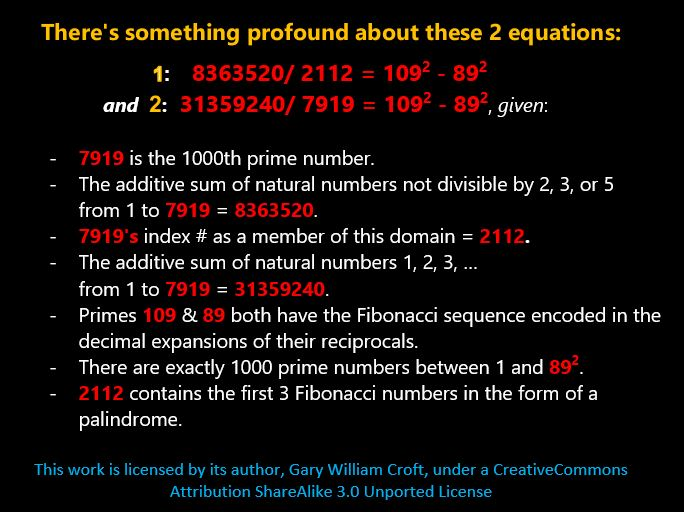Then, consider that the first 24 prime numbers, 2 + 3 + 5 + 7 + 11 + 13 + 17 + 19 + 23 + 29 + 31 + 37 + 41 + 43 + 47 + 53 + 59 + 61 + 67 + 71 + 73 + 79 + 83 + 89 = 963 = 3 * 321, while the average value of the 2112 elements in our domain from 1 to the 1000th prime number, 7919 = 3960 = 1092 − 892 = 11 * 360. And 11 plus its digital root = 13. Not only are 11 and 13 the first twin prime pair in our domain but 11 + 13 = 24, the digital root periodicity of our domain, and 242 = 576, the number of modulo 90 factorization dyads and rotating {9/3} star polygons that determine the positioning of all composite numbers within our domain. Given the pervasiveness of palindromic prime 11 and its multiples in our schema, it would be fair to call it the 'Fundamental Repunit.' It's the axe blow that splits a log perfectly down the middle with one fell swoop.

We've waxed 'much ado' above and elsewhere on this site about the mathematical 'object' we argue encapsulates the first 1000 prime numbers. Here's a link to a page dedicated entirely to this conjecture: The Mystery of the First 1000 Prime Numbers

Period 24 In All Directions

"24 hours in a day and 24 beers in a case. Coincidence? I think not!” – H. L. Mencken

We stated earlier that this is a 24-dimension model. After putting in the proverbial 10,000 hours studying '24-beat' patternization, we've come to the conclusion that period-24 is the key to the "Theory of Everything" and that a hypothetical E24 Petrie Projection will one day loom large as E8 is understood to be the leg of a triad, with E16, leading to E24; the three being analogous to:

Mod 30 → E8 → {3} star polygon ...

Mod 60 → E16 → {6/2} star polygon ...

Mod 90 → E24 → {9/3} star polygon ...

... building geometrically to infinity ...

We've dubbed this 'The Theory of Everything ... but the Kitchen Sink,' illustrated below:Explore the incredible symmetries that come into focus when the lense aperature, so to speak, of the Prime Spiral Sieve is tripled to modulo 90, synchronizing its modulus with its period-24 digital root, and perhaps you'll see why we make this bold assertion. The mathematical balancing and resolution of this domain, which correlates with a hypothetical E24, including structures that determine the distribution of prime numbers, are fundamentally period-24.

Aside from each of the 24 deterministic modulo 90 factorization progressions having a repetition cycle of 24, there is a hidden dimension generated by successive digital root dyads for each factorization sequence that is also period-24 given that (and there's a proof for this): Any initial dyad of non-zero single-digit numbers enters a periodic cycle of length-24 (all of which have additive sum = 117; although interestingly sum to 99 when 9's are 'cast out' as is the convention in digital root math), except for eight pairs that enter cycles of length-8, {(3,3), (3,6), (3,9), (6,3), (6,6), (6,9), (9,3), (9,6)}, and one dyad, (9,9), which is periodic of length-1. (Source: Adapted from a comment posted on the On-Line Encyclopedia of Integer Sequences: OEIS #A030132: Digital Root of Fibonacci(n)). For example, the first two terms of the Fibonacci digital root sequence just referenced, (1,1), enter a periodic cycle of length-24, as follows:

{1, 1, 2, 3, 5, 8, 4, 3, 7, 1, 8, 9, 8, 8, 7, 6, 4, 1, 5, 6, 2, 8, 1, 9} {repeat ... }.

The matrix below demonstrates how the twenty-four period-24 cycles can be networked to construct a geometrically expandable triangle with palindromic reflectional symmetry that stairsteps the Fibonacci digital root sequence bidirectionally from the ground up: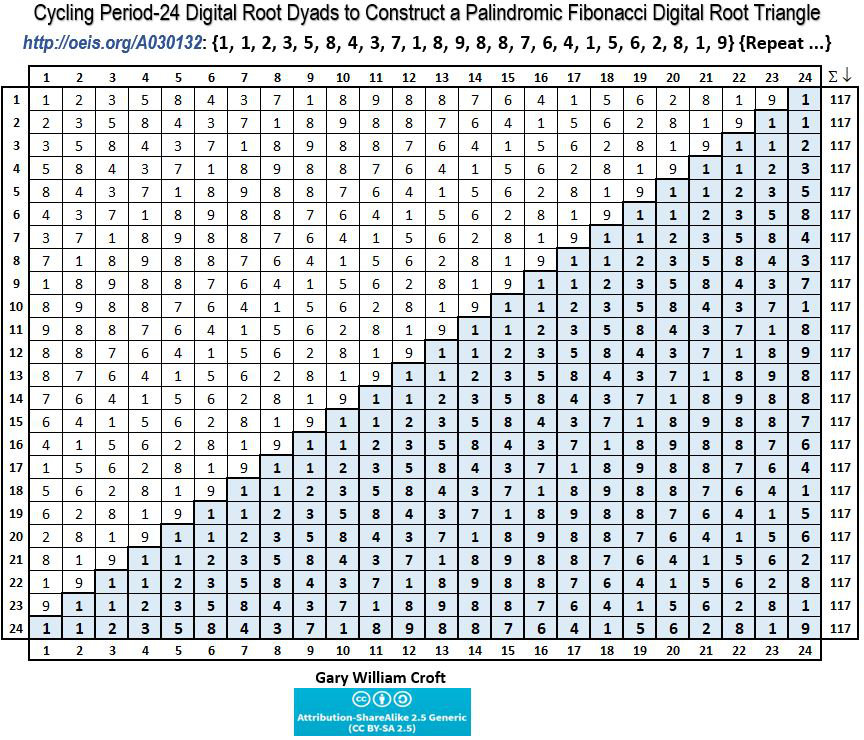Our modulo 90 framed domain, n ≌ to {1, 7, 11, 13, 17, 19, 23, 29, 31, 37, 41, 43, 47, 49, 53, 59, 61, 67, 71, 73, 77, 79, 83, 89} mod 90, also has a period-24 digital root:

{1, 7, 2, 4, 8, 1, 5, 2, 4, 1, 5, 7, 2, 4, 8, 5, 7, 4, 8, 1, 5, 7, 2, 8} {repeat ...}.

The twenty-four period-24 digital root factorization sequences derived from our domain can be parsed into six (6) digital root sub-sets, each consisting of 4 elements, that roll up under digital roots 1, 2, 4, 5, 7, 8, as follows (noting that all sequences for the first complete factorization round initialize with n x 7; the 1st and 2nd rounds are demarcated by 912 and the 2nd round initializes with 91 x 97. Further down this page you'll find a link to a spreadsheet with tables that make these sequences and their transitions clear.):

Numbers ≌ {1, 19, 37, 73} mod 90 (i.e., digital root = 1) sequence as:
{7, 2, 4, 8, 1, 5, 2, 4, 1, 5, 7, 2, 4, 8, 5, 7, 4, 8, 1, 5, 7, 2, 8, 1} {repeat ... }
Numbers ≌ {11, 29, 47, 83} mod 90 (i.e., digital root = 2) sequence as:
{5, 4, 8, 7, 2, 1, 4, 8, 2, 1, 5, 4, 8, 7, 1, 5, 8, 7, 2, 1, 5, 4, 7, 2} {repeat … }
Numbers ≌ {13, 31, 49, 67} mod 90 (i.e., digital root = 4) sequence as:
{1, 8, 7, 5, 4, 2, 8, 7, 4, 2, 1, 8, 7, 5, 2, 1, 7, 5, 4, 2, 1, 8, 5, 4} {repeat … }
Numbers ≌ {23, 41, 59, 77} mod 90 (i.e., digital root = 5) sequence as:
{8, 1, 2, 4, 5, 7, 1, 2, 5, 7, 8, 1, 2, 4, 7, 8, 2, 4, 5, 7, 8, 1, 4, 5} {repeat … }
Numbers ≌ {7, 43, 61, 79} mod 90 (i.e., digital root = 7) sequence as:
{4, 5, 1, 2, 7, 8, 5, 1, 7, 8, 4, 5, 1, 2, 8, 4, 1, 2, 7, 8, 4, 5, 2, 7} {repeat … }
Numbers ≌ {17, 53, 71, 89} mod 90 (i.e., digital root = 8) sequence as:
{2, 7, 5, 1, 8, 4, 7, 5, 8, 4, 2, 7, 5, 1, 4, 2, 5, 1, 8, 4, 2, 7, 1, 8} {repeat … }

Take a closer look at the above sequences, and you'll detect the perfect bilateral 9-sum and diagonal 90-sum symmetry hi-lited below:The color-coded matrices pictured below illustrate that, when you align the twenty-four period-24 digital root sequences, perfect symmetry is revealed, i.e., every row has 12 bilateral 9 sums working from the outside in (or inside-out), and the additive sum of every row and column is 108. The principal diagonals also have 12 bilateral 9 sums, albeit in a cross-diagonal manner, summing to 75 + 141 = 216 = 108 x 2.And now to examples. Below are shown two of the 24 sets of period-24 digital root factorization sequences we introduced earlier, i.e., numbers congruent to {7} and {11} modulo 90 sequentially multiplied times numbers congruent to {1, 7, 11, 13, 17, 19, 23, 29, 31, 37, 41, 43, 47, 49, 53, 59, 61, 67, 71, 73, 77, 79, 83, 89} modulo 90, starting with 7. In addition to modulo 90, we display modulo 30 patterns and the interesting way they parse with repeating bilateral-30 sum symmetries. To the right of each digital root sequence we demonstrate how they generate rotating triangulations ({1,4,7}, {2,5,8}, {3,6,9}) in the form of 3 x 3 matrices which in turn can be extrapolated into {9/3} star polygons: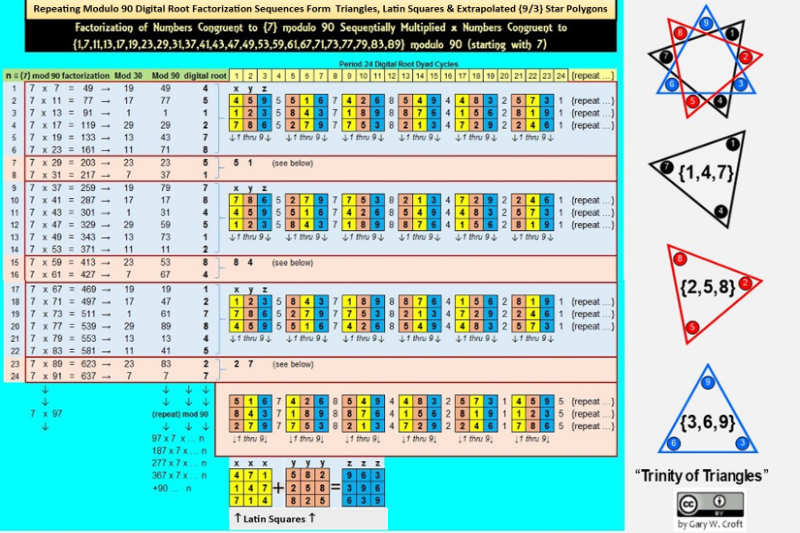Scanning the factorization products in the two examples above, we can quickly determine which of them impact twin prime candidates, given all twin primes greater than (5,7) are congruent to either {11,13}, {17,19} or {29, 1} modulo 30. For example, for n congruent to {7} modulo 90 factorization configurations pictured above, we see that exactly 18 of the 24 products (or 75%), index (identify) composite twin prime candidates (shown in brackets): 47 , , 79, 89 ,  121, 131 ,  163, 257 ,  289, 299 ,  331, 341 ,  373, 467 ,  499, 509 ,  541, 551 , and  583. Note how the lower vs. higher valued products alternate with each pair of twin prime candidates.

The remaining 6 products, of course, identify "isolated" prime candidates that are composite. We go into greater depth regarding digital root dyad cycles specifically related to twin primes on our page devoted to twin primes under the headings "Digital Root Factorization Dyads of the Twin Prime Distribution Channels" and "Twin Prime Magic Squares."

To access an Excel spreadsheet showing the derivation of all 24 digital root factorization sequences and their corresponding period-24 matrix cycles, click here: Digital_Factorization_Roots_24x24_Matrix.xlsx.

Tables within the afore-linked spreadsheet reveal how the 24 infinitely repeating period-24 sequences each generates 24 matrices that extrapolate to {9/3} star polygons for a total of 24 x 24 = 576 = 9 x 82 9-pointed star polygons per complete factorization cycle. [Note: This count excludes the 'Fibo Bounds' described in the Note following this paragraph.] Also revealed are beautiful mod 30 vs. mod 90 symmetries. For example, comparing the digital root sequences for numbers congruent to {1} vs. {29} mod 30 and/or {1} vs. {89} mod 90 exposes beautiful counter-balancing symmetries.

[Note: Numbers ≌ {1} modulo 90 squared are segregated from the sets of 24x24 factorization sequences. Namely, 1x1, 91x91, 181x181, ... create a 'supra-sequence' of demarcating squares directly before and after each of the complete factorization sets of 24. For example, 1x1 and 91x91 act as bookends before 1x7 and after 89x91 (which in turn draws a line before 91x97). Poetically–if not profoundly–numbers ≌ {1} modulo 90 squared have other dimensions of 'oneness' when you consider that their digital roots and terminating digits also equal One.]

We'll close this section with a complex polygon constructed from the period-24 digital root vectors of our domain framed modulo 90: